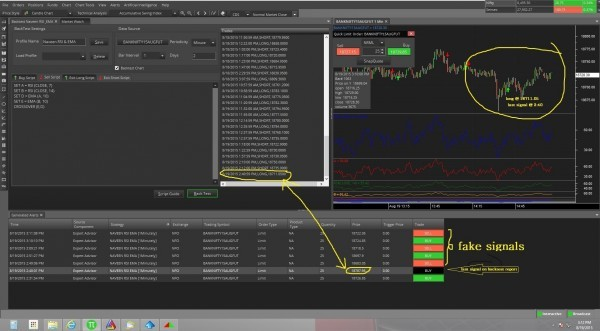# Expert advisor (Relative Strength Index with EMA) give fake generated alerts,Relative Strength Index with EMA EA gives fake generated alerts , i have doubt and checked with backtest . there is no signals on the charts ,... how to trade with EA in this condition ....

As per the above query in EA the RSI with EMA the code is given below

```SET A = RSI(CLOSE, 7)
SET B = RSI(CLOSE, 14)
SET C = RSI(CLOSE, 21)
SET D = EMA(A, 10)
SET E = EMA(B, 10)
CROSSOVER(D,E)```

Sell Script:

```SET A = RSI(CLOSE, 7)
SET B = RSI(CLOSE, 14)
SET C = RSI(CLOSE, 21)
SET D = EMA(A, 10)
SET E = EMA(B, 10)
CROSSOVER(E,D)```

the alerts comes according to the above code.

Dear GEEK ALGO
i have tested above code which gives alert when the crossover happen …
but my question is .above code generated alerts doesnot match with the backtested alerts …
both signals are diffrent in price & time …